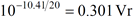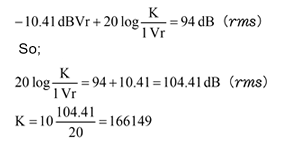The unit calibration compensation value to display the sound pressure level unit (dB), when the MI-8100 directional microphone is used with the FFT Analyzer.When the MI-8100 directional microphone is combined with SR-2200 sensor amplifier, and used with CF-7200 portable FFT Analyzer, the unit calibration compensation value is necessary to display the value as the unit of sound pressure level (dB) with the CF-7200. The explanation is as follows.

 Measurement condition   • MI-8100 sensitivity: -30.41 dB re. 1 V/Pa at 1 kHz (From the laboratory data attached to MI-8100.) • SR-2200 gain setting: 20 dB (-10 to 60 dB, 10 dB-step eight switching) If the MI-8100 is connected directly to the CF-7200 without using the SR-2200, please set the gain value of the SR-2200 into "0 dB"

Sound pressure level (dB) is effective value. On the other hand, the time waveform which is outputted by the MI-8100 via SR-2200 is not effective value but instantaneous value. When the voltage output from the SR-2200 is analyzed by FFT analyzer, Y axis of FFT is displayed to be dB effective value, and the signal is correctly displayed as the pressure level (dB) by using the unit calibration function.

MI-8100 is the directional microphone, so the measurement value is different from the non-directional microphone such as a sound level meter.

Calculation method of unit calibration compensation value

When the MI-8100 is used with the SR-2200, conversion from output voltage (V) to sound pressure level (dB) is considered as follows. The "r" of "Vr" means effective value in the following explanations.

MI-8100 directional microphone sensitivity

When sensitivity of the MI-8100 "-30.41dB re.1 V/Pa at 1 kHz" means "When the frequency is 1 kHz and sound pressure is 1 Pa (sound pressure level: 94 dB), the output voltage becomes -30.41 dBVr (voltage)".

SR-2200 microphone amplifier output voltage

When the gain of the SR-2200 is set as 20 dB, the output voltage of SR-2200 is 20 dB (10 times) amplified voltage of MI-8100. So, when the sound pressure is 1 Pa (sound pressure level: 94 dB), the output voltage is -30.41 + 20 dB = -10.41 dBVr. When the gain of the SR-2200 is 20 dB, the voltage for sound pressure 1 Pa is -10.41 dBVr. Convert it into the voltage as follows;Therefore, when frequency is 1 kHz and sound pressure is 1 Pa, the voltage output from the SR-2200 is 0.301 Vr.

Compensation value of CF-7200 portable FFT Analyzer unit calibration

When 1 Pa is defined as 94 dB, the following formula is established. When the unit calibration compensation coefficient is considered as K;The power spectrum can be displayed as sound pressure level unit (dB) by setting unit calibration of CF-7200 as "166149 EU/V".

Revised:2008.10.20